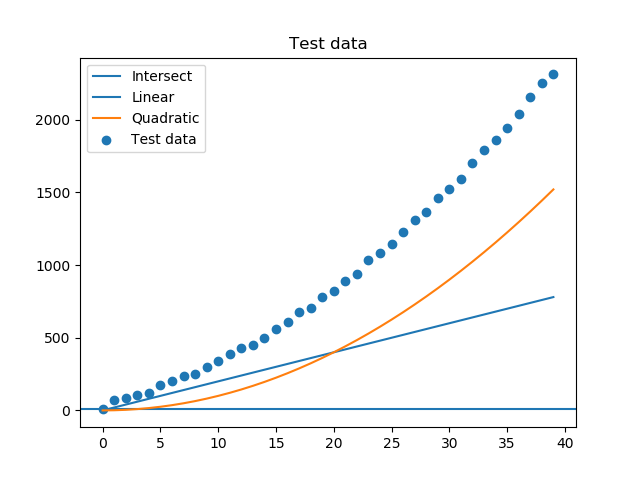# Feature engineering in python

What: Generating features for regression models in python based on formulas (like in R)
Why: Model notation in formulas and not in code => Better maintainability
How: Use patsy

# Test data

Lets take as example a simple: fitting an exponential to some test data. First, some test data needs to be generated:

```import numpy
import pandas

length = 40
linear = numpy.array(range(length))*20
quadratic = numpy.array([e**2 for e in range(length)])
intersect = 10
random = numpy.random.random(length)*40

df = pandas.DataFrame(
{
"y":  linear + quadratic + intersect + random,
"x1": range(length)
})
```

The test data and its components look like this:# Feature engineering

Lets assume, we want to fit the data with a linear and quadratic term (surprise, surprise). When using a linear model, you would provide a matrix where each column is one of the features. In our case this would mean writing a small script to fill a length x 3 matrix. Although it is no big deal it involves some lines of code and is not very readable.

With patsy we can write instead something like the following and let the matrices be generated by patsy:

```1 2 3 import patsy formula = "y ~ x1 + numpy.power(x1, 2)" y, x = patsy.dmatrices(formula, df)```

This will result for x in something like:

```[[1.000e+00 0.000e+00 0.000e+00]
[1.000e+00 1.000e+00 1.000e+00]
[1.000e+00 2.000e+00 4.000e+00]
[1.000e+00 3.000e+00 9.000e+00]
[1.000e+00 4.000e+00 1.600e+01]
...
```

# Fitting

With sklearn it is easy to do the fit:

```1 2 3 4 import sklearn.linear_model model = sklearn.linear_model.LinearRegression() model.fit(x, y) predicted = model.predict(x)```

In the end, it looks like: